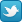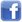Sign in Register
search | recent topics | hottest topics
How to solve this one?Author Message
shiro

Joined: 8 Nov 2014
Messages: 1
Offline

What is next logical move?This message was edited 1 time. Last update was at 15 Nov 2014 12:15:26Stickywulf

Joined: 22 Nov 2013
Messages: 2
Offline

shiro wrote:What is next logical move?Look at Row 5 Column 9 (R5C9). It must be either 1 or 4. If we pretend that it does equal 4 then we find out quickly that this can't be true. Follow the logical steps below:

Let R5C9 = 4
Then R4C8=1
Then R7C8=9
Then R7C2=1
Then R5C2=4 .... but R5C2 can't equal 4 since we let R5C9 equal 4.

Therefore R5C9 can't be 4, and so R5C9=1

This message was edited 1 time. Last update was at 16 Nov 2014 05:38:26rdwells

Joined: 29 Jul 2013
Messages: 1
Offline

shiro wrote:What is next logical move?Check out R1C3. If that's a 6, then there are 4 squares in a rectangle (R2C3, R2C5, R3C3, and R3C5) that are all either 1 or 4; this would lead to multiple solutions, which would mean the puzzle is invalid. So R1C3 must be 4.

Question for others: Is the assumption that there are no multiple solutions valid? It should be true of any well-created puzzle, and I use often it in situations like the one here, but it just kinda feels wrong.GreenLanternJoined: 28 Nov 2014
Messages: 4
Offline

A Unique Rectangle at r23c35 allows us to conclude that either r2c3=6 or r3c3=6 and therefore, r1c3<>6, and the puzzle is solved.

Some solvers like to avoid using uniqueness assumptions in their solution, but I don't see the point in that since by definition, a valid sudoku must have a single unique solution. Why limit the number of solving techniques in your toolbox?jeka

Joined: 12 Jul 2010
Messages: 263
Offline

rdwells wrote:
Check out R1C3. If that's a 6, then there are 4 squares in a rectangle (R2C3, R2C5, R3C3, and R3C5) that are all either 1 or 4; this would lead to multiple solutions, which would mean the puzzle is invalid. So R1C3 must be 4.

If you keep solving the puzzle with this assumption, i.e. that R1C3 is 6, you will come to a point where you'll realize that, in fact, no solution exists, i.e. putting a 6 in R1C3 is wrong. So, no, this will not lead to multiple solutions, but rather to no solution at all.

rdwells wrote:
Question for others: Is the assumption that there are no multiple solutions valid? It should be true of any well-created puzzle, and I use often it in situations like the one here, but it just kinda feels wrong.

This assumption is valid, however, this approach is still wrong.

Now about solving this particular puzzle, notice that R7C2 and R8C9 will always have the same value (either a 1 or a 9) that is enforced by R7C8, however, that value cannot be 1 because that would prevent both R5C2 and R5C9 from having a 1. Therefore, the value in both R7C2 and R8C9 must be a 9.

This message was edited 1 time. Last update was at 1 Dec 2014 22:53:59GreenLanternJoined: 28 Nov 2014
Messages: 4
Offline

Actually, I was too hasty. r1c3<>6 only advances the puzzle to this point:And here, the puzzle is finally solved by making another uniqueness deduction. This is a BUG+1 grid which allows us to immediately conclude r8c2=1, and the puzzle is reduced to singles.Go to:  Select a forum Sudoku Cut and Roll Eggs Lines Website Other